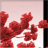# Calculate Z Values Using CalculateField_management

2536
2
08-13-2012 11:32 AMNew Contributor
Hi,

I'm wanting to calculate Z values from a PointZM shapefile that I created using the 3D analyst extension InterpolateShape. I know that you can just right click from within Arc and calculate the geometry using the z value but I wanted to do this using geoprocessing.

Currently I've got:

`arcpy.CalculateField_management([InputPointZ], "METERS", "!shape.Z!", "PYTHON_9.3", "")`

I don't know what the proper syntax for calculating the Z value and I'm assuming it's something like
`"!shape.Z!"`
, but that's not it. Anyone been able to do this with the CalculateField_management tool?
Tags (3)
2 RepliesRegular Contributor II
Basically your approach isn't going to work exactly, as you have to alter the row's geometry object.

Going to need to set !shape!.Z here, so maybe do it as a side effect of calculating some other field. For example, an expression of

`CalcZ(!METERS!, !shape!)`

and a codeblock of

```def CalcZ(meter_length, shapeval):
shapeval.Z = 100
return meter_length
```

This would recalculate METERS to itself and as a side effect change the Z value to 100. Though this all feels like a somewhat indirect approach, you are likely much better off in just writing a short python script with an UpdateCursor as it'd be a lot more coherent and not many more lines of code.

```cur = arcpy.UpdateCursor(InputPointZ)
for row in cur:
row.shape.Z = 100
rows.updateRow(row)

del row, cur```byEsri Regular Contributor
Jason W.

Have you considered running the Add XY Coordinates tool?  If the input is Z aware it will return the Z value from the Shape field as an output attribute.

Best,
Eric